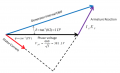# Synchronous machine voltage problem, thanks

#### epicfrank

Joined May 26, 2013
5
Hi,

I am a student doing a electrical machine course and i was given a question which says

"A 200kVA, 660V, 50Hz, 4-pole star-connected synchronous generator has a synchronous reactance of 1 ohm per phase. When the machine is operating at lagging power factor, rated voltage and current, the torque angle δ=tan-1(0.2). Calculate the power factor and torque
"

The 660V means the line voltage right? In the answer there is this diagram:I am not sure should the phase voltage be calculated by 660/root3=381.1V because the phase voltage is the voltage of the load end and there is a armature reactance on the way, shouldn't the 381.1v be the generator internal EMF in the triangle?